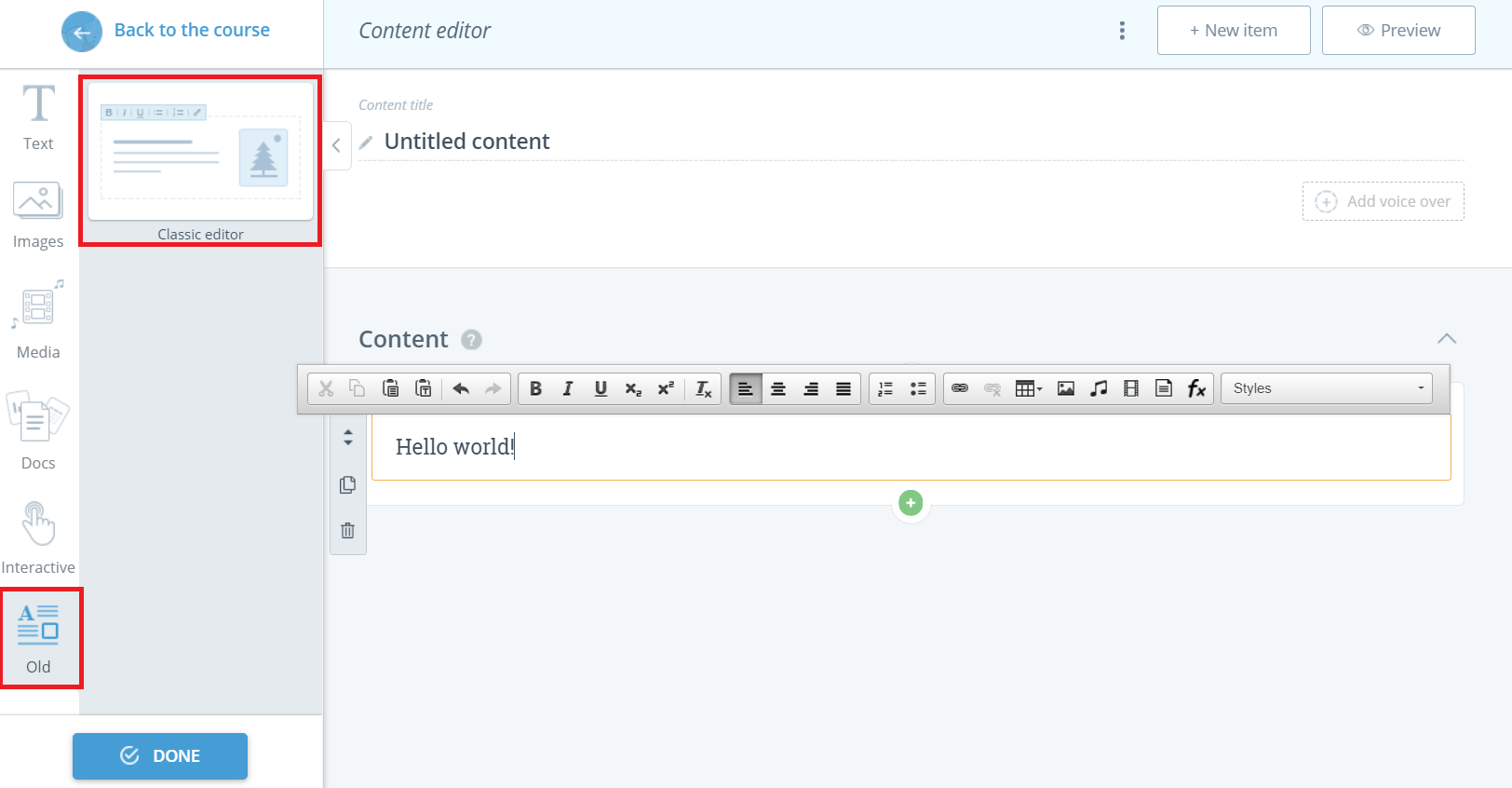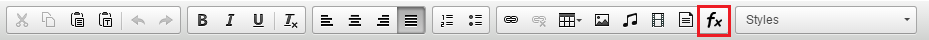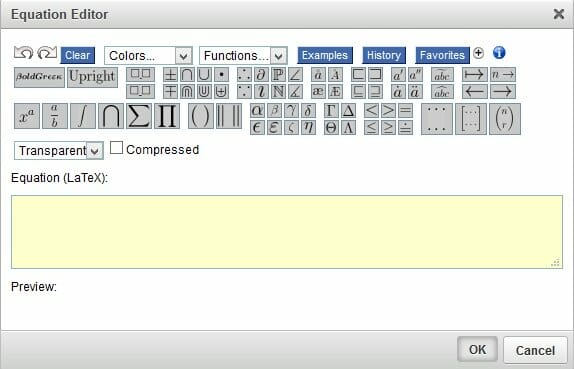Insert an equation

Using the Equation Editor, you can create mathematical equations and add them to any content area of your course.

Written Olga Kuzmenko

Using the Equation Editor, you can create mathematical equations and add them to any content area of your course.

To create an equation, you need to add a content page, choose the Old editor and select the Classic editor block.Click within the text area where you need to add it and then click on the Insert Equation button.Now you can create your equation.Double-click an existing equation to edit it.

Suggested articles: